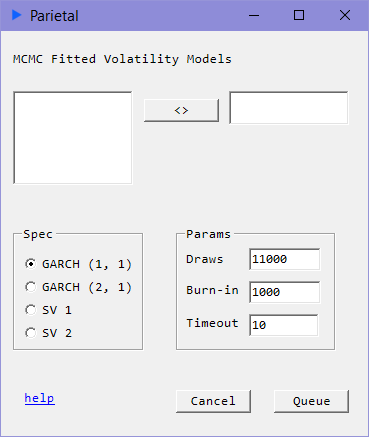# GARCH MCMC

## Time Series Volatility#### Description#

Fitting GARCH models via maximum likelihood estimation can sometimes be a bit tricky. Here we offer an alternative using markov chain monte carlo for GARCH(1, 1) and GARCH(2, 1) models.

#### Model: GARCH(1, 1)#

$$y_{t} = \mu + \epsilon_{t}, \epsilon_{t} \sim N(0, \sigma_{t}^{2})$$ $$\sigma_{t}^{2} = \alpha_{0} + \alpha_{1} \epsilon_{t-1}^{2} + g_{1} \sigma_{t-1}^{2}$$

#### Model: GARCH(2, 1)#

$$y_{t} = \mu + \epsilon_{t}, \epsilon_{t} \sim N(0, \sigma_{t}^{2})$$ $$\sigma_{t}^{2} = \alpha_{0} + \alpha_{1} \epsilon_{t-1}^{2} + g_{1} \sigma_{t-1}^{2} + g_{2} \sigma_{t-2}^{2}$$

### Input#

• draws: Run the sample this many times.
• burn-in: Discard these many samples from the beginning draws. Burn-in must be less than draws.
• timeout: Time limit in minutes.

### Returns#

• coef: Coefficient estimates for $g$ for GARCH parameters and $a$ for ARCH parameters
• serr: Standard Errors
• $\sigma_{t}$: volatility process